Function Repository Resource:

# NumberTheoreticTransform

Compute the number-theoretic transform of a list of integers

Contributed by: Andrew Ortegaray
 ResourceFunction["NumberTheoreticTransform"][{f1,f2,…},r,m] finds the number-theoretic transform of the integer list fi with the given root r and modulus m. ResourceFunction["NumberTheoreticTransform"][{f1,f2,…},m] finds the number-theoretic transform of the integer list fi with given modulus prime m and automatically chosen root r. ResourceFunction["NumberTheoreticTransform"][{f1,f2,…}] finds the number-theoretic transform of the integer list fi with automatically chosen root r and modulus m.

## Details

The number-theoretic transform (NTT) Fj of an integer list fi of length n with root r and modulus m is defined to be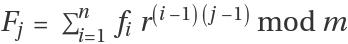.
ResourceFunction["NumberTheoreticTransform"] to a list of integers is analogous to Fourier for a list of reals.
For a NTT of a list fi of length n to support convolution with prime modulus m, the m must be of the form kn+1 for some integer k. The root r must then be chosen to be an nth root of unity modulo m.
For a NTT of a list fi of length n to support convolution with composite modulus m in general: (1) n must possess a ModularInverse modulo m, (2) r must be an nth root of unity modulus m, (3) (rm-1) is relatively prime to m for all integers m from 1 to m-1.
There are other equivalent conditions that can be chosen to establish the convolution property of an NTT.
ResourceFunction["NumberTheoreticTransform"][] is compatible with lists of any length less than 212, however, all lists of length 212 or greater must be a power of two, due to practical speed constraints.
If only the modulus is given in addition to fi, then it must be chosen to be prime and of the form kn+1.
The initial use of ResourceFunction["NumberTheoreticTransform"] may be slow due to one-time compilation of functions. Subsequent uses will execute quickly.

## Examples

### Basic Examples (3)

The number-theoretic transform of the list {1,2,3} can be computed with modulus 7 = 2 × 3 + 1 and root PrimitiveRoot2:

 In:=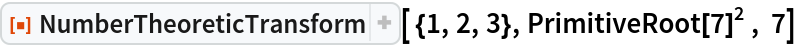Out=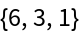The number-theoretic transform of the list Range can be computed with modulus 31 = 10 × 3 + 1 and root PrimitiveRoot10:

 In:=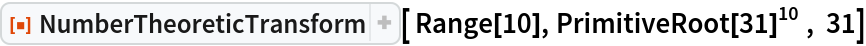Out=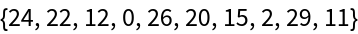The number-theoretic transform of the list Range can be computed with modulus 4129 and root 1163:

 In:=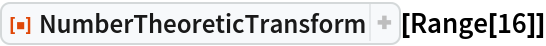Out=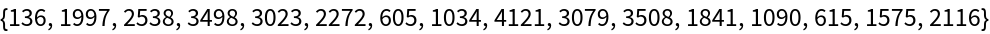### Properties and Relations (4)

The Inverse of a number-theoretic transform is a transform with the same modulus m, but with root ModularInverse[r,m] and prefactor ModularInverse[n,m]:

 In:=Out=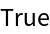Number-theoretic transforms can be used immediately to compute exact convolutions of non-negative integer lists:

 In:=Out=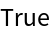In:=Out=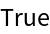Number-theoretic transforms can be used to compute exact convolutions of general integer lists, including negative integers. However, an offset correction needs to be computed first:

 In:=Out=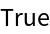Number-theoretic transforms can be computed much faster on lists with lengths that are powers of two:

 In:=Out=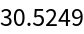### Possible Issues (2)

If the chosen modulus m is too small, then overflow may cause the number-theoretic transform to have no inverse:

 In:=Out=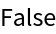If the chosen modulus m is too small, then overflow may cause the number-theoretic transform to not support convolutions:

 In:=Out=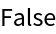### Neat Examples (1)

Using convolution with padding, number-theoretic transforms can compute polynomial multiplications:

 In:=Out=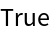## Publisher

Wolfram Summer School

## Version History

• 1.0.0 – 10 February 2021